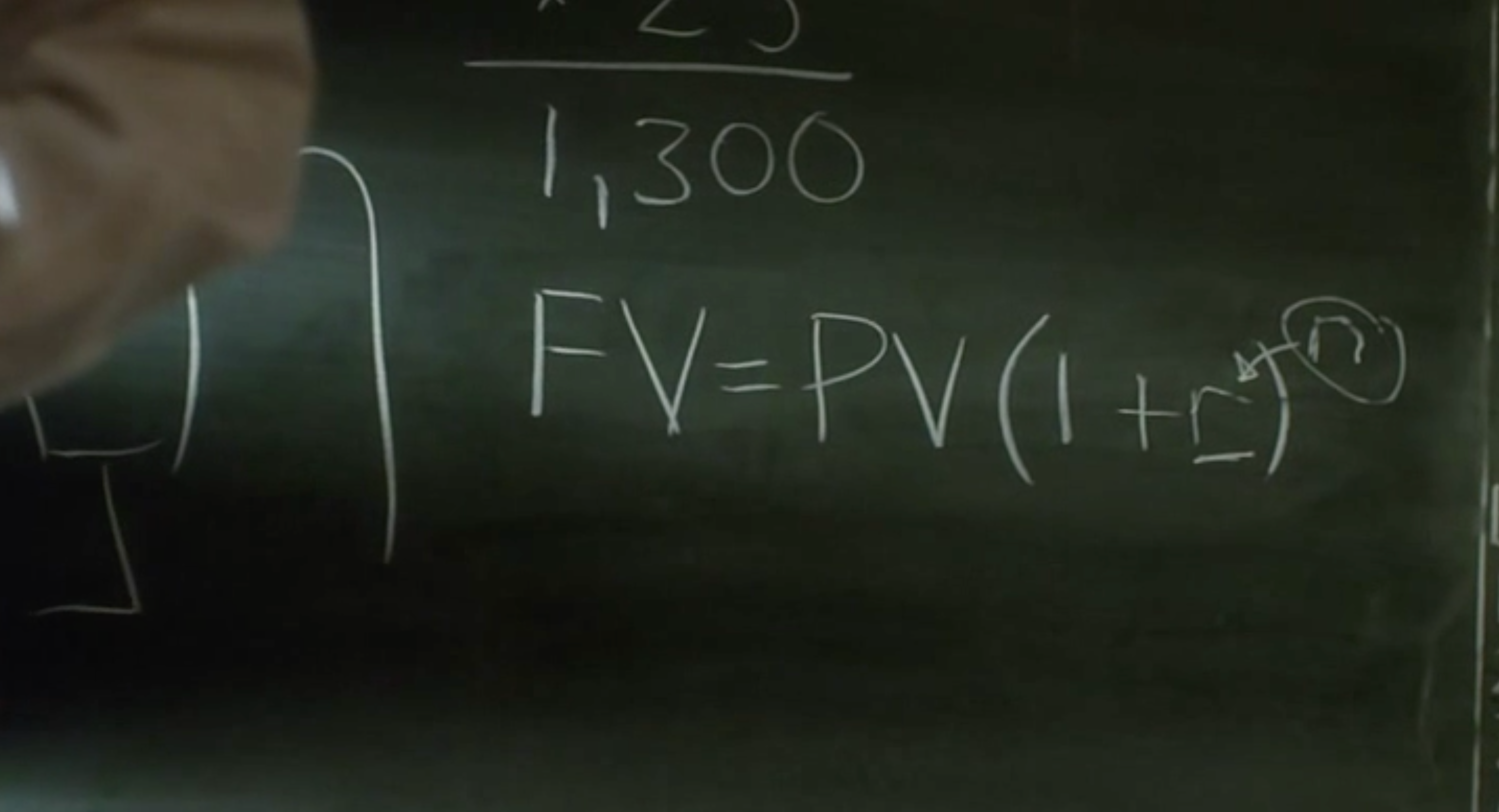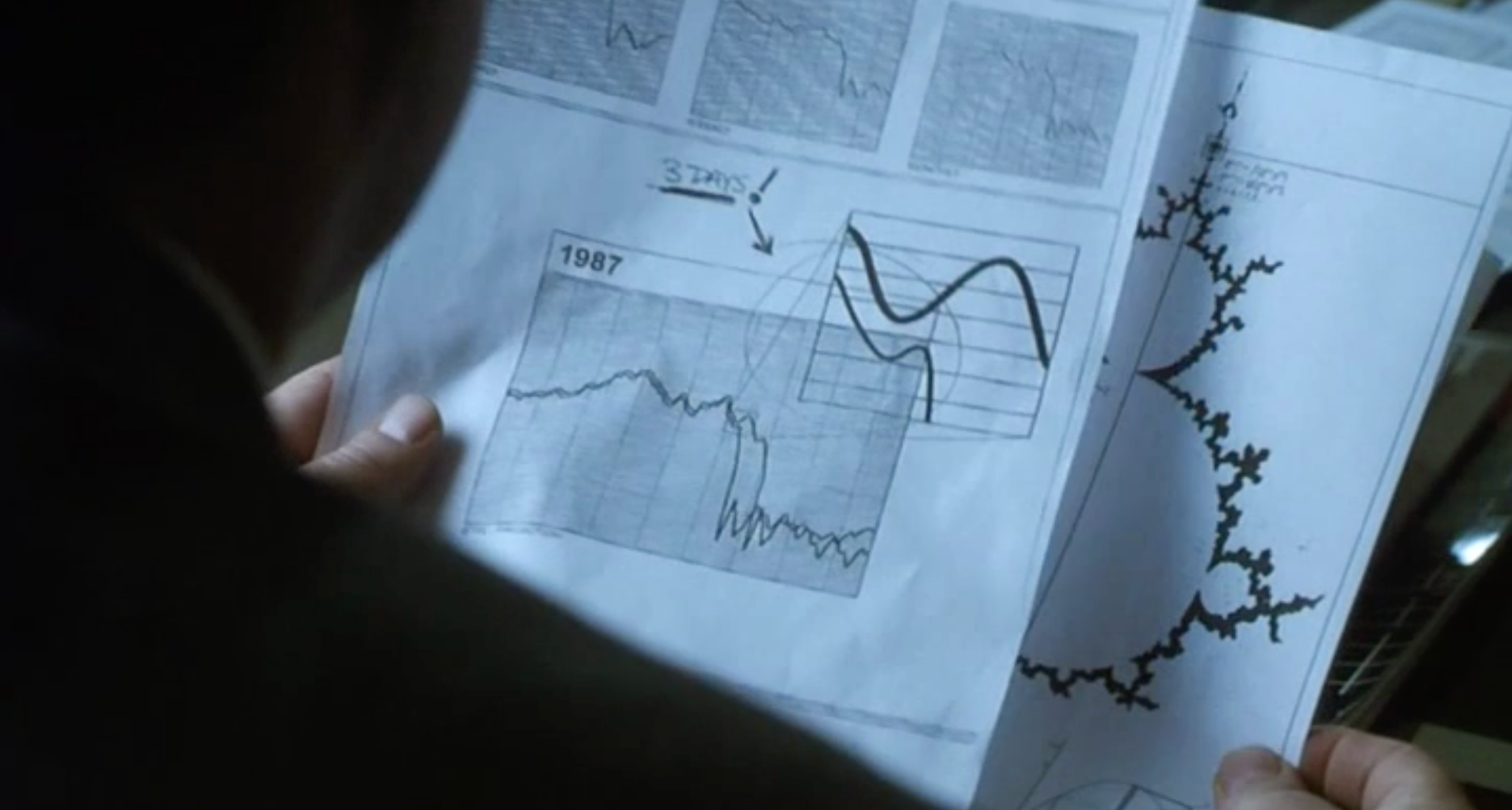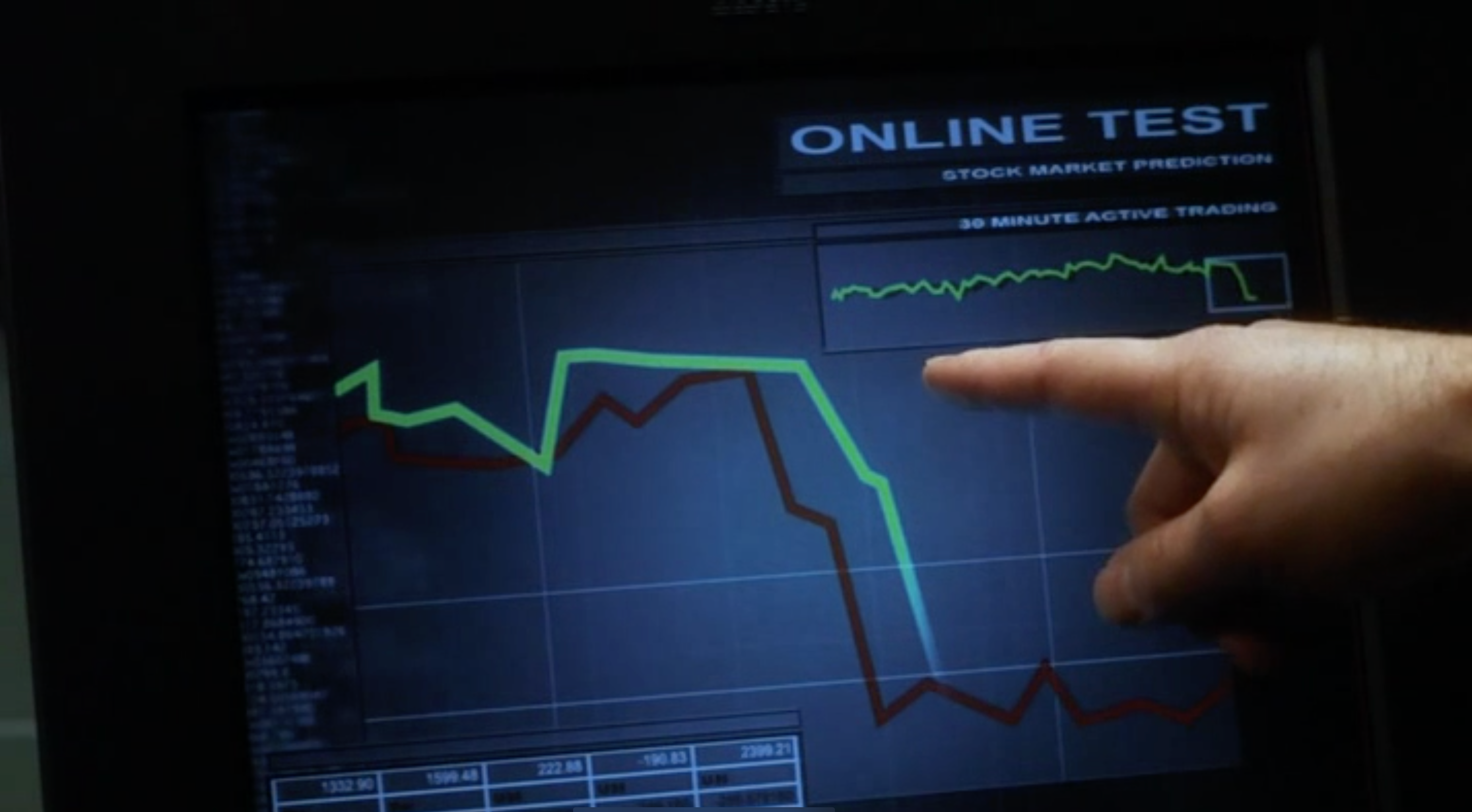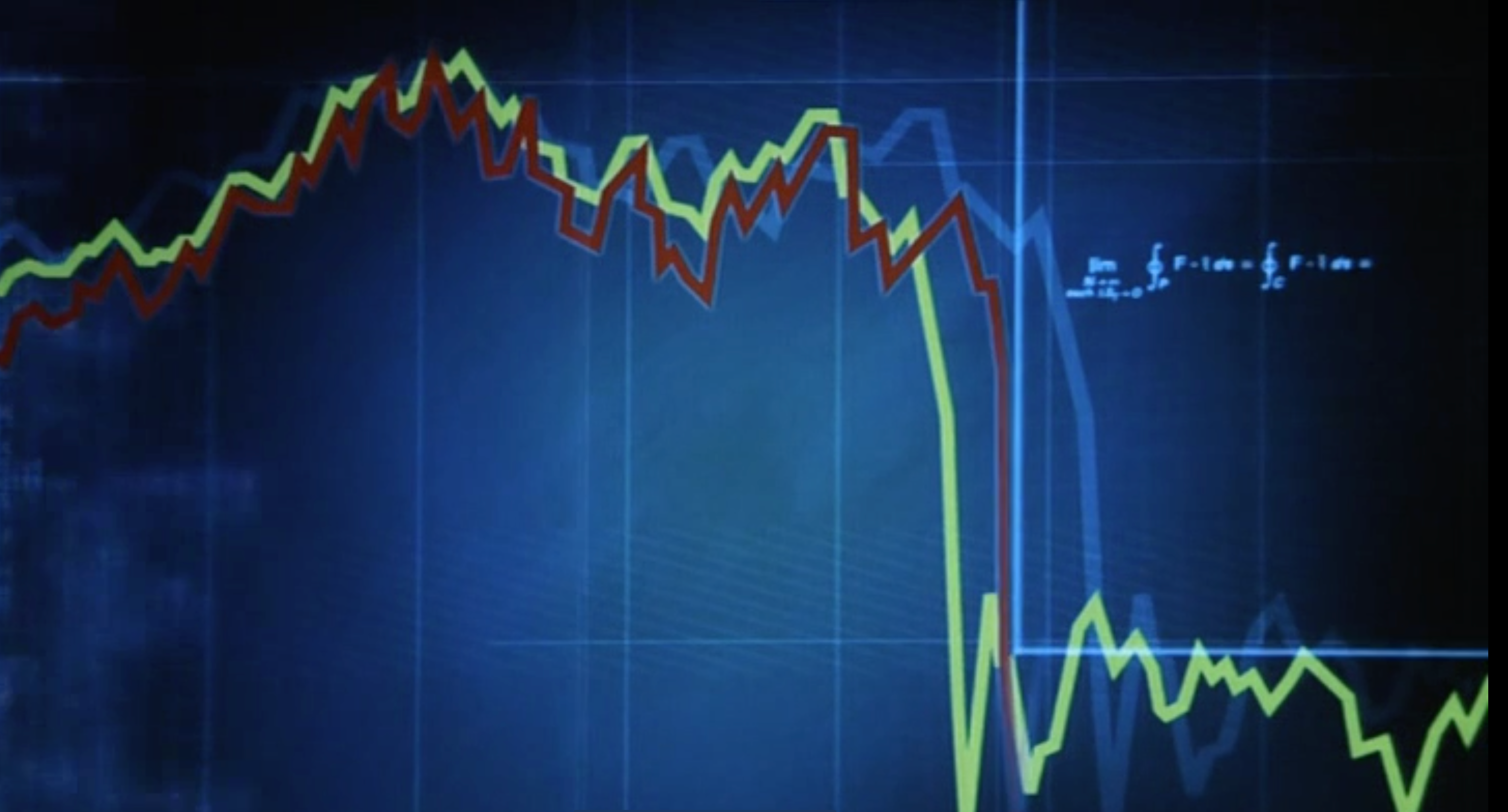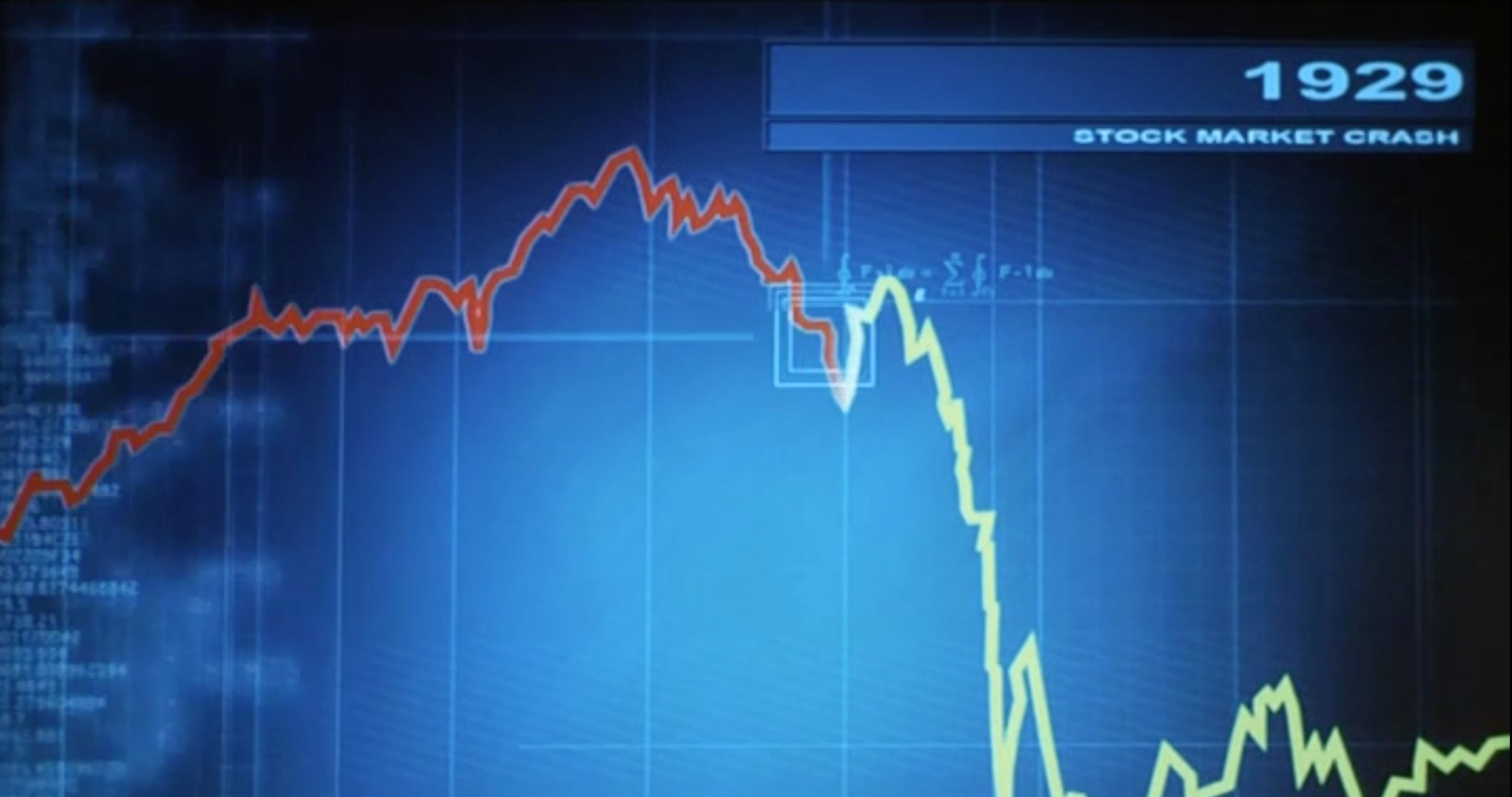# The bank (2001)We had that movie already in the math in movie collection but in a bit smaller resolution. The movie features the Mandelbrot set and also the compound interest formula. Let me comment on this again: even so it has appeared in this Pecha Kucha Talk of mine from 2013. Lets for a positive h define (1+h)n where n is the closest integer to x/h. In banking, h is the interest rate and n is the number of years. In physics, h might be the Planck constant, a very small number and n counts a distance x in terms of the number of Planck constants which have to be stacked together. The magic is that if h goes to zero, then the compound interest function f(x) = (1+h)n = (1+h)x/h converges to the exponential function exp(x). The most popular definition of exp(x) is to see it as a limit of polynomials (1+x/n)n. The compound interest version f(x) = (1+h)x/h has the advantage that one can immediately see that f(x+h)=(1+h) f(x) so that (f(x+h)-f(x)/h = f(x). This is an exact relation for the average rate of change on an interval of length h. One can also just (as described in that Pecha-Kucha talk) be more radical and just deform the entire calculus we know to the discrete. It requires to deform the polynomial algebra so that one still has all the relations from calculus like d/dx xn = n xn-1 or d/dx exp(x) = exp(x). The nice thing about this quantum deformation of calculus is that we do not have to change the textbooks. If done properly, all relations we see in these books hold. One consequence however is that the cosine and sine functions are not perfectly periodic any more. They grow in amplitude. But the effect is small. A gamma ray starting at the time of the Big Bang only becomes stronger in amplitude when reaching us after billions of years. We would see gamma ray bursts.

M4V, Ogg Webm. imdb link,